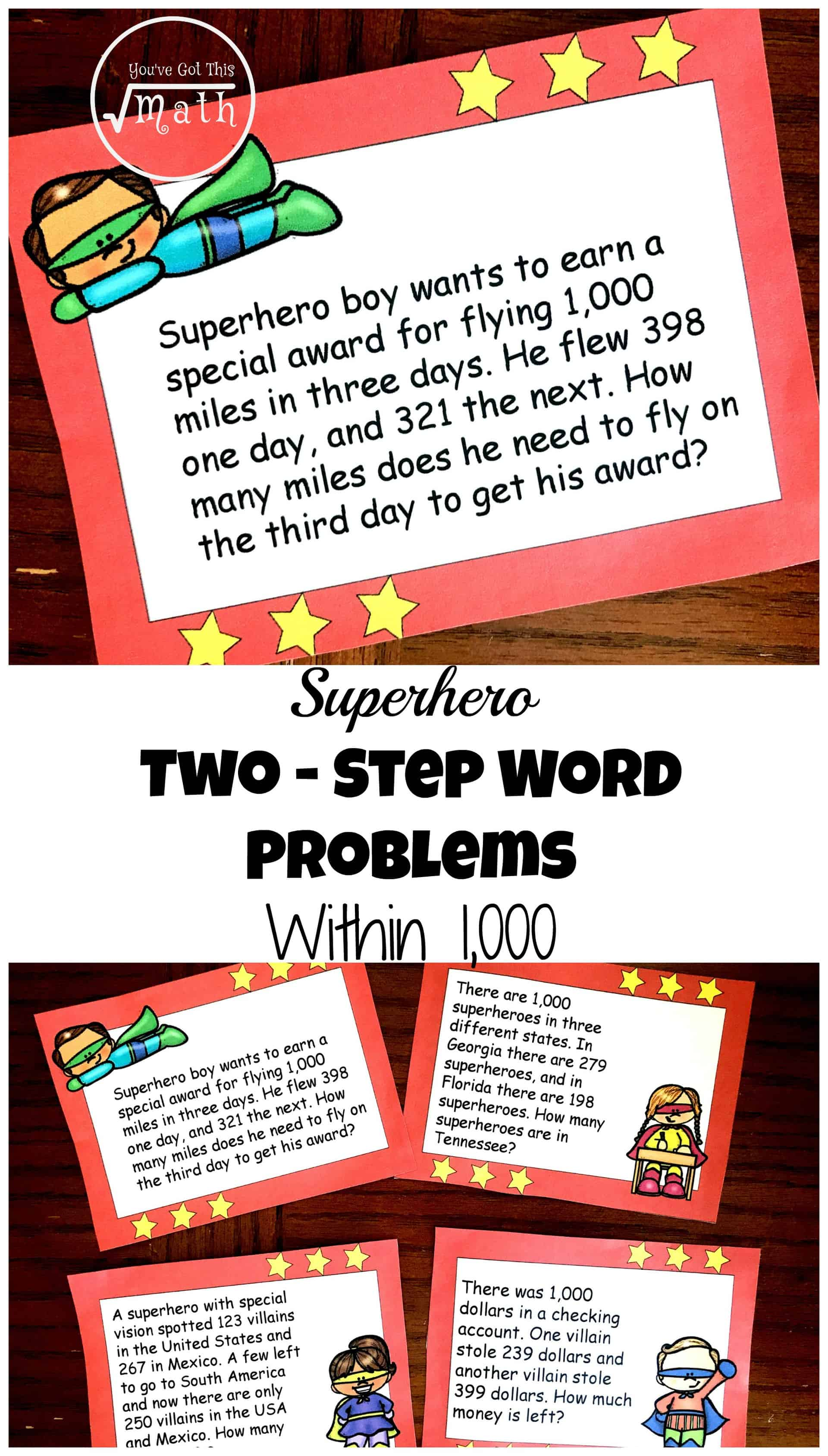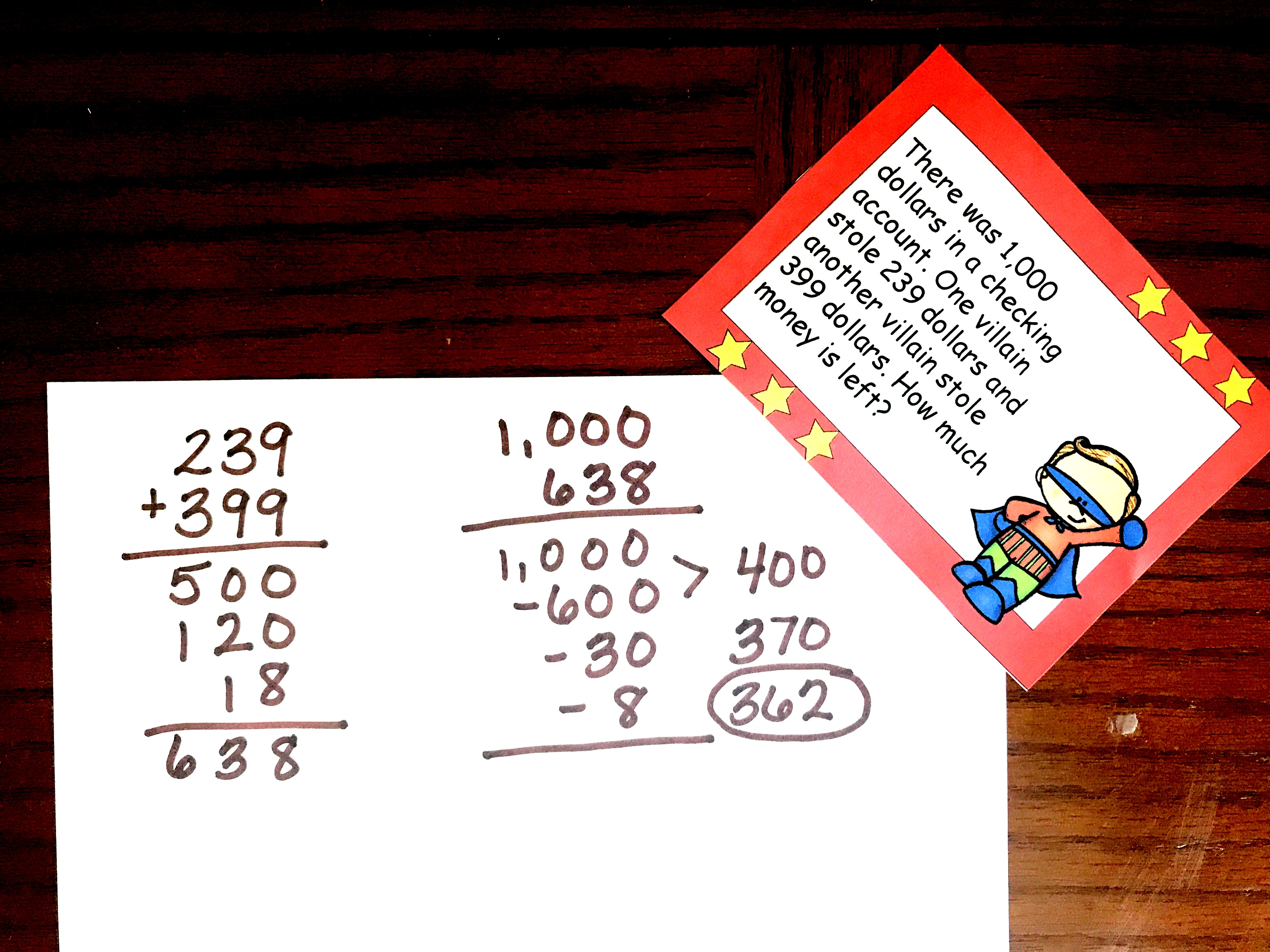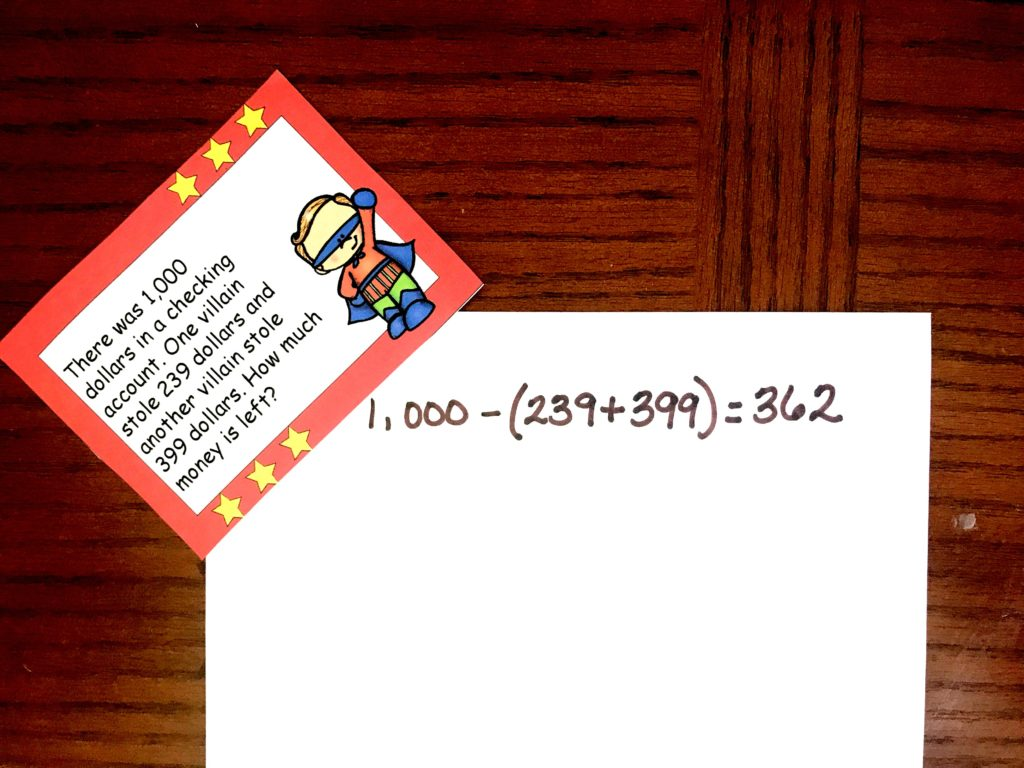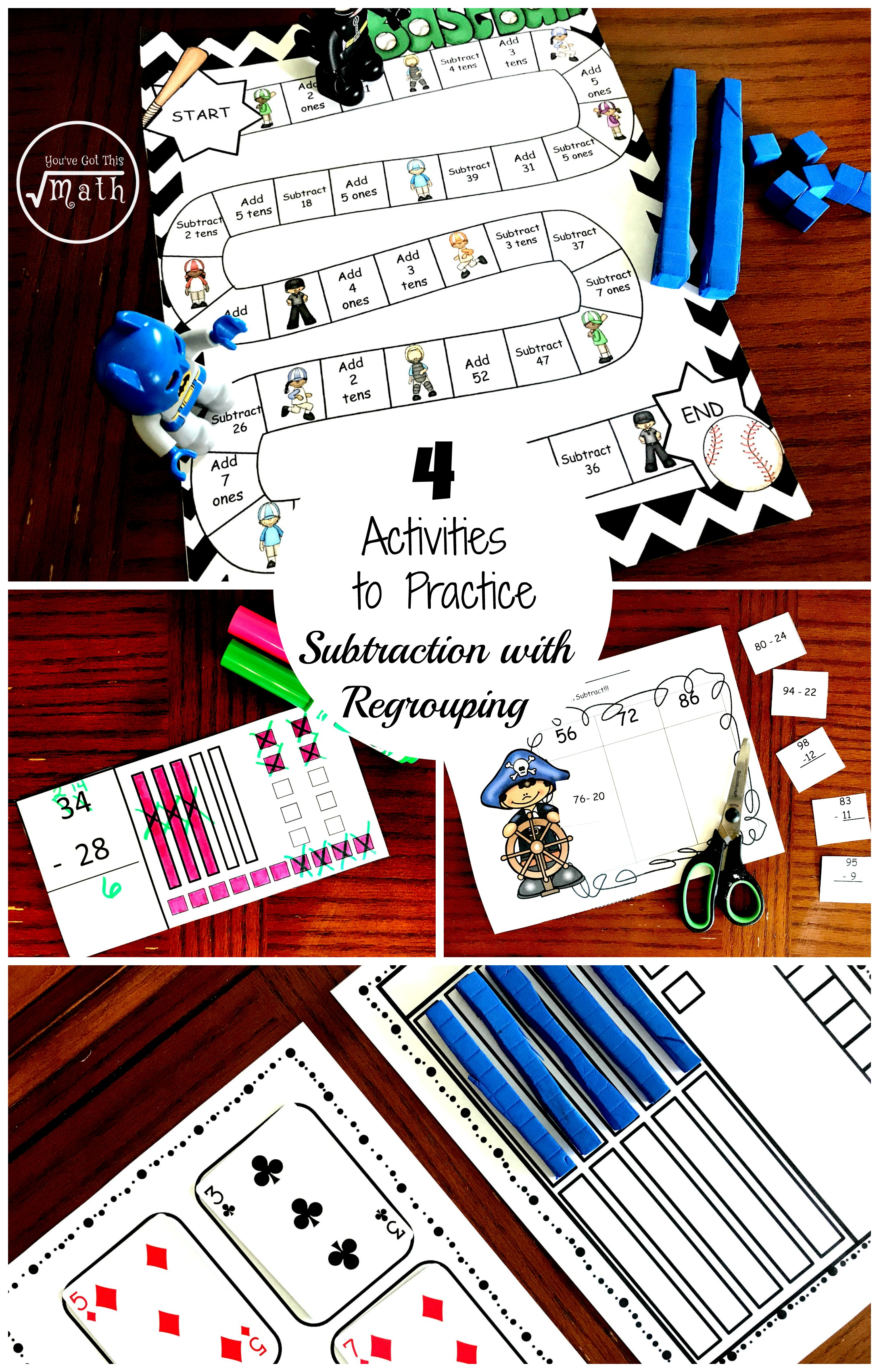Home » Math » 12 FREE Superhero Two-Step Word Problems Worksheets or Task Cards

# 12 FREE Superhero Two-Step Word Problems Worksheets or Task Cards

Grab these superhero two-step word problems worksheet that focus on adding and subtracting within 1000. The fun superhero theme adds excitement to the task.

### You can get your free printable at the bottom of the post by clicking on the large blue button.Mine was solving two at the end of a page of practice. You know….I just completed 20 subtraction problems and then I had to do two word problems that you guessed it, had to do with subtraction.

There was very little instruction on how to do word problems, or even figuring out which operation I should do.

Nowadays there is a greater focus on solving word problems and completing them on their own.

### Teaching Word Problems

There are many strategies for teaching word problems, but I love This Reading Mama’s word problem sort as a way help children focus on the vocabulary in word problems. It is a great place to start.

Next, I’m a big component of modeling over and over again what you expect when your students are solving a word problem.

Finally, I love having children share how they solved their word problems. The simple explanation of a child may make sense to a child that is struggling to understand.

### What I Expect With Word Problems

When my children are solving word problems I expect three things..and sometimes I add in a fourth.

• Modeling

There are many ways to model a word problem. A student can draw base ten blocks, use a number line, or even ten frames.In this example, the child decided to use a number line. They started at 1,000 and then subtracted 500 since 200 + 300 = 500. Next, you can see that they subtracted another 100 and two tens since s0 + 90 = 120. Their next step was to subtract another 10 and then 8 since 9 + 9 = 18. Finally, they ended up 362.

• Show your work

We are always asking children to do this. And when we teach word problems separately and provide opportunities for them to see us solve them and how others solved them, they are more likely to do it.In this example, the child used partial products to solve the problem and their work is clearly laid out.

• Equation

We know Algebra is basically work in solving equations. When we have our children write out equations for word problems, we are laying a foundation for Algebra.This child created this equation for the work they did. But there are other ways you could write one. If you have multiple students doing these word problems, this is another great place to share all the different equations your students came up with.

### Prep – Work For Superhero Two-Step Word Problems Worksheets

To help students get practice in word problems, I created these Superhero Two-Step Word Problems Worksheets or Task Cards.  They allow children to focus on word problems and not just complete them at the end of a worksheet.

There are two way you could use them.

Option 1:

• Print off the Two-Step Word Problems Within 1,000 on card stock paper and laminate
• Puch a hole in them and place them on a ring
• Put the ring in a math center and have the children solve one a day in their math journals
• Make sure you model your expectations first and then have children share their models and equations.

Option 2:

• Print off the Two-Step Word Problems Within 1,000 on regular paper
• Have the children glue the word problem into their math journals
• Have all your children solve one word problem a day.
• Make sure you model your expectations first and then have children share their models and equations.

Word problem practice is important. I hope these word problems allow you to easily focus on this concept.

You’ve Got This

Rachel

You may also be interested in: